#### Denseness

Consider constructing an infinite sample sequence over. What would be some desirable properties for this sequence? It would be nice if the sequence eventually reached every point in, but this is impossible becauseis uncountably infinite. Strangely, it is still possible for a sequence to get arbitrarily close to every element of(assuming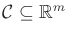). In topology, this is the notion of denseness. Let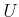and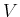be any subsets of a topological space. The setis said to be dense inif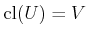(recall the closure of a set from Section 4.1.1). This means adding the boundary points toproduces. A simple example is that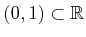is dense in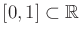. A more interesting example is that the set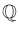of rational numbers is both countable and dense in. Think about why. For any real number, such as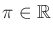, there exists a sequence of fractions that converges to it. This sequence of fractions must be a subset of. A sequence (as opposed to a set) is called dense if its underlying set is dense. The bare minimum for sampling methods is that they produce a dense sequence. Stronger requirements, such as uniformity and regularity, will be explained shortly.

Steven M LaValle 2020-08-14